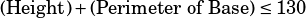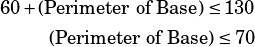Test 7 Section 3 #14

OK, so in this question we’re told that this company restricts package sizes such that the height of the box plus the perimeter of the base of the box cannot exceed 130 inches. The words “cannot exceed” can be translated to “is less than or equal to.” A peek at the answer choices shows that each one has a “0 <” at the beginning (because boxes can’t have negative dimensions), but to keep our work simple for now we’ll just incorporate that at the end.Then we’re told that we have a box with a height of 60 inches and an unknown base perimeter. So the first step here is to recognize that we’ve got 70 inches to play with for the base perimeter.Now let’s deal with the perimeter. The perimeter of a shape is the sum of the lengths of its sides. For a rectangular box with width w and length l, the perimeter will be 2w + 2l. In this particular case, we’re told that the width is and the length is 2.5x, so the perimeter should be 2x + 2(2.5)x, which can be simplified.Now include the “0 <” we discussed up top and you’re at choice A.